#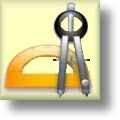Geometry Worksheets

## Angles Worksheets for Practice and Study

Here is a graphic preview for all of the Angles Worksheets. You can select different variables to customize these Angles Worksheets for your needs. The Angles Worksheets are randomly created and will never repeat so you have an endless supply of quality Angles Worksheets to use in the classroom or at home. We have classifying and naming angles, reading protractors and measuring angles, finding complementary, supplementary, verical, alternate, corresponding angles and much more. Our Angles Worksheets are free to download, easy to use, and very flexible.

These Angles Worksheets are a great resource for children in 3rd Grade, 4th Grade, 5th Grade, 6th Grade, 7th Grade, and 8th Grade.

Click here for a Detailed Description of all the Angles Worksheets.

## Quick Link for All Angles Worksheets

Click the image to be taken to that Angles Worksheets.

##### Classifying AnglesAngles Worksheets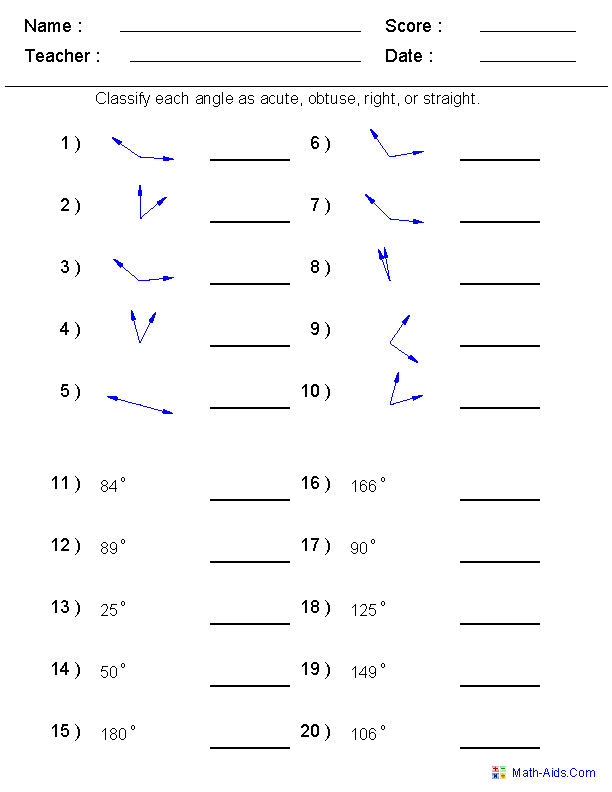##### Naming AnglesAngles Worksheets##### Angle Pair RelationshipsAngles Worksheets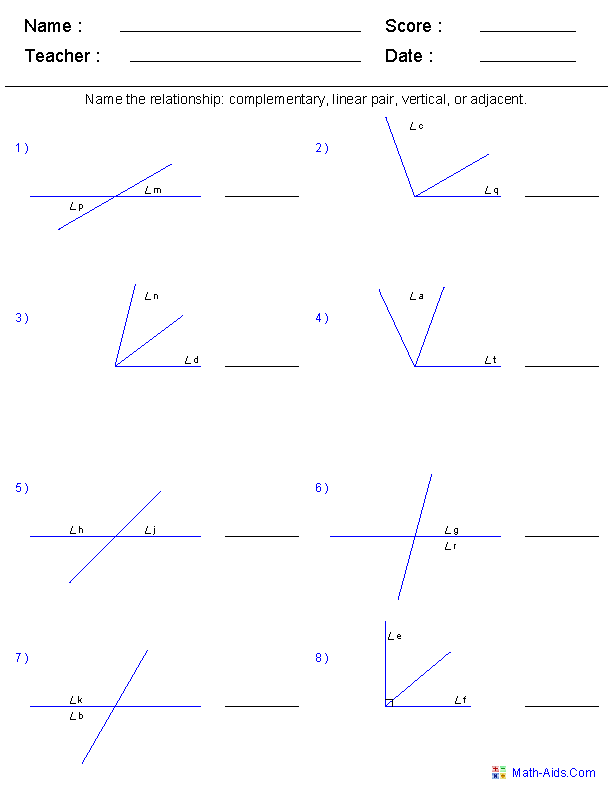##### Producing Protractor ImagesAngles Worksheets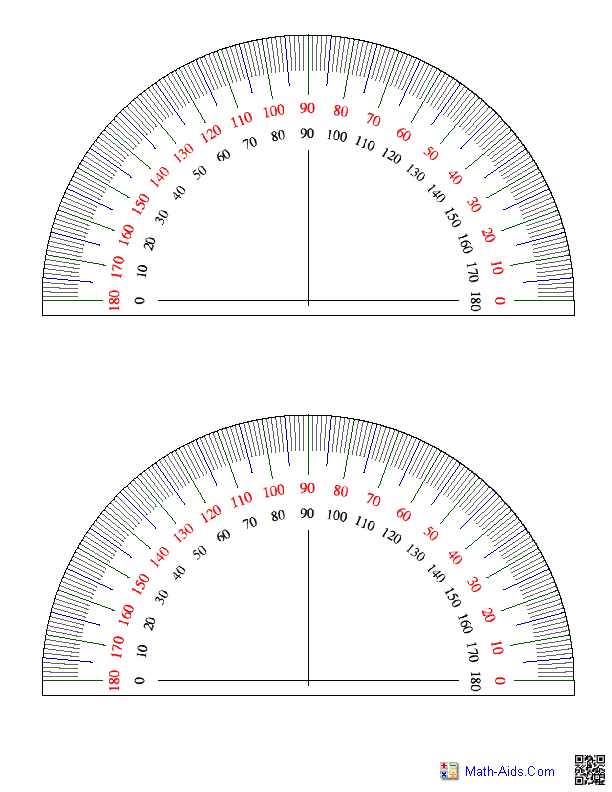##### Reading a ProtractorAngles Worksheets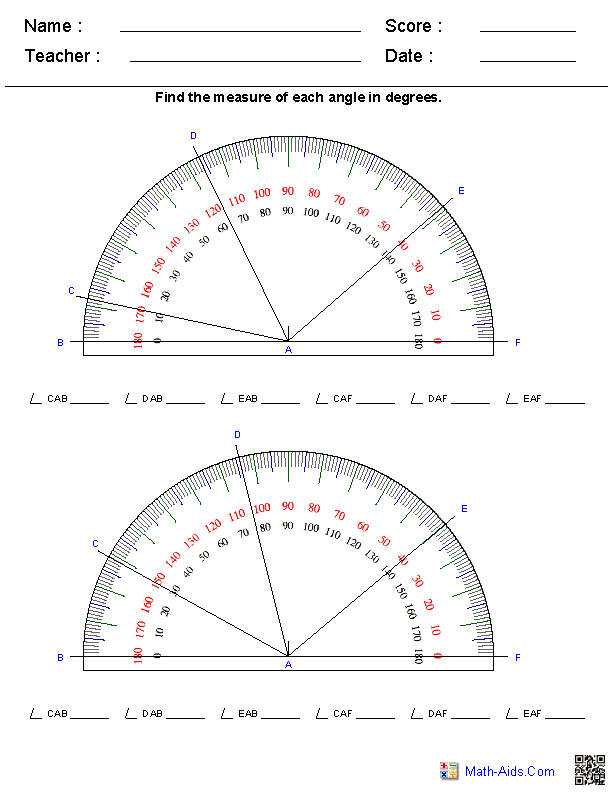##### Measuring AnglesAngles Worksheets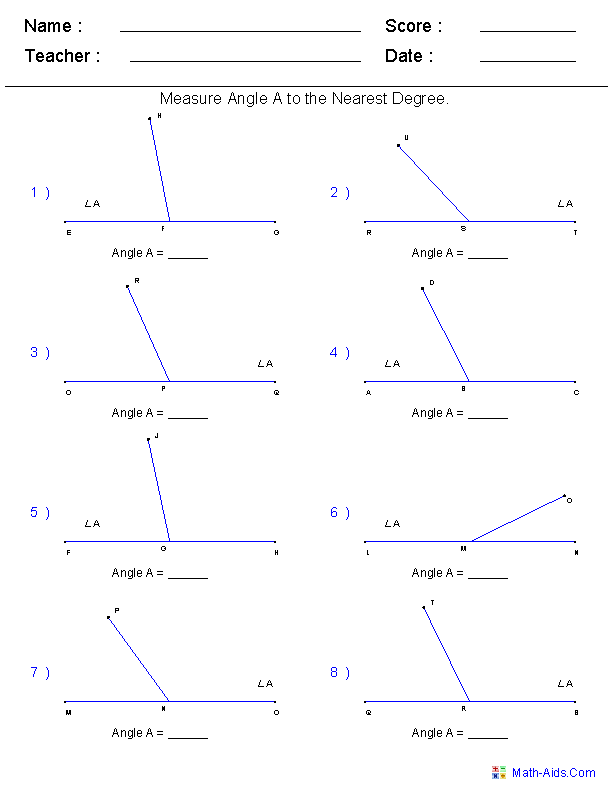##### Drawing AnglesAngles Worksheets##### Identify if a Point is Interioror Exterior to an Angle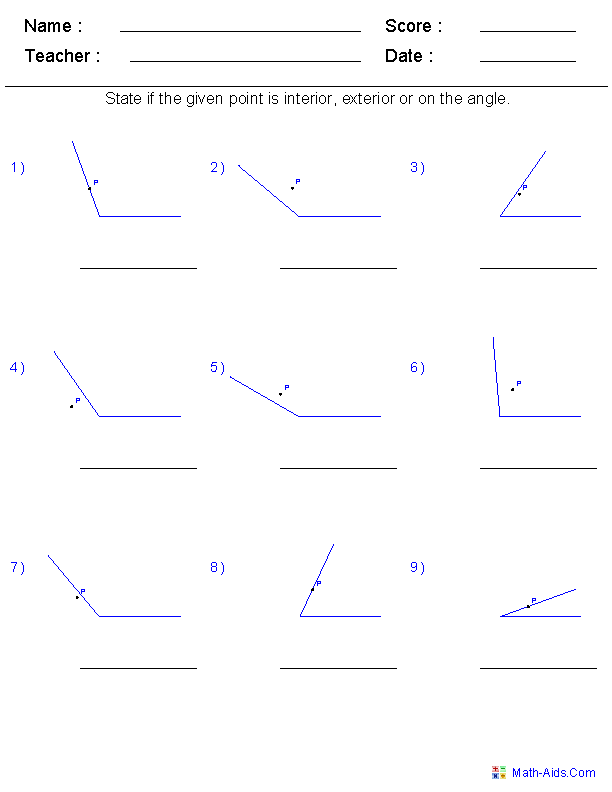##### Angle Addition PostulateAngles Worksheets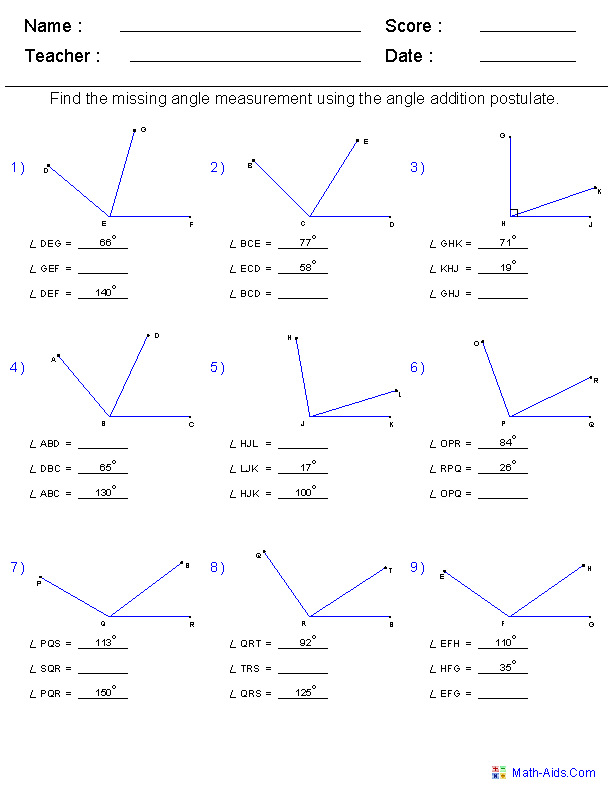##### Find Complementary AnglesAngles Worksheets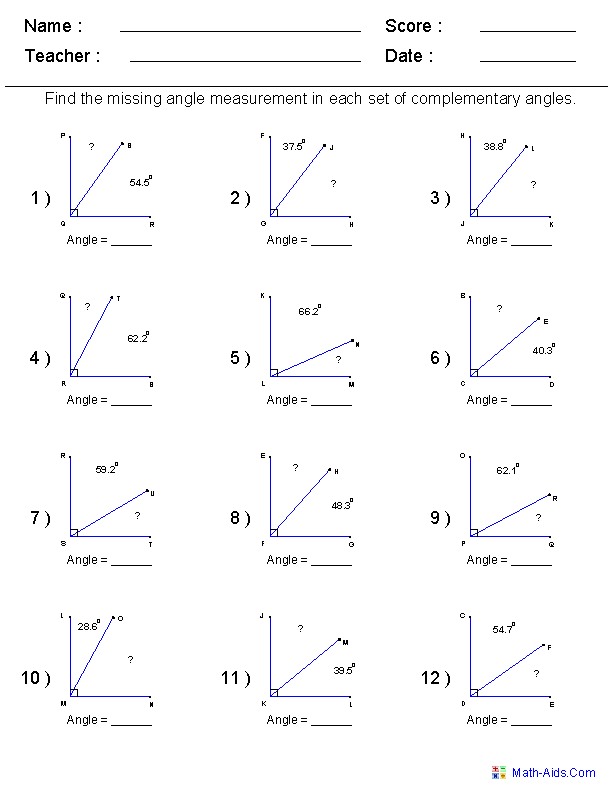##### Find Supplementary AnglesAngles Worksheets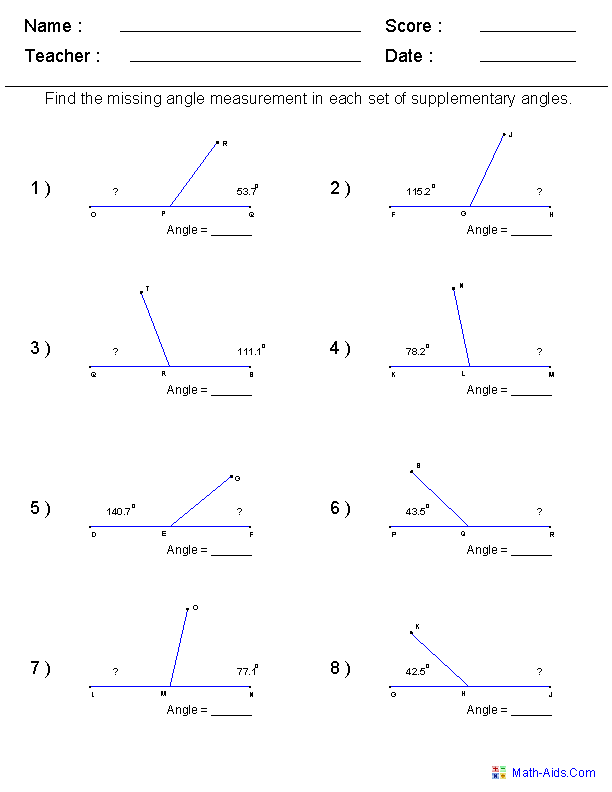##### Find Vertical AnglesAngles Worksheets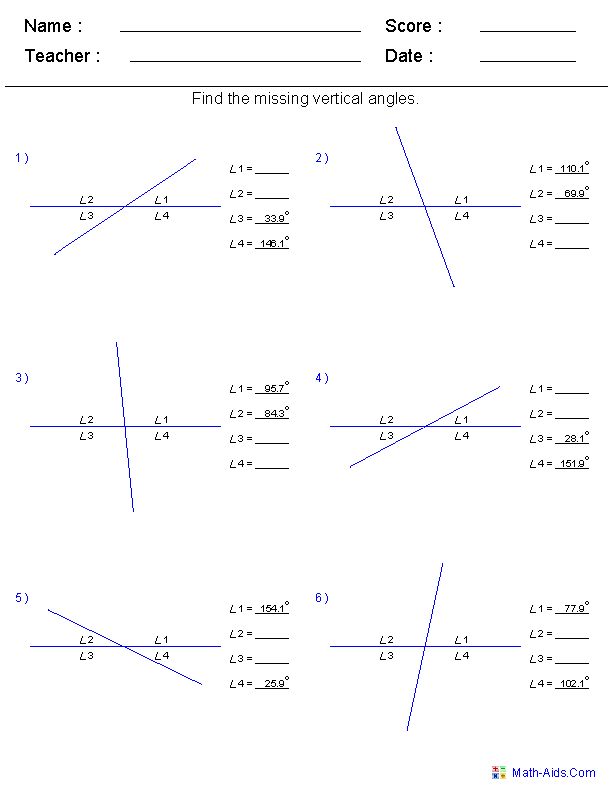##### Find Alternate AnglesAngles Worksheets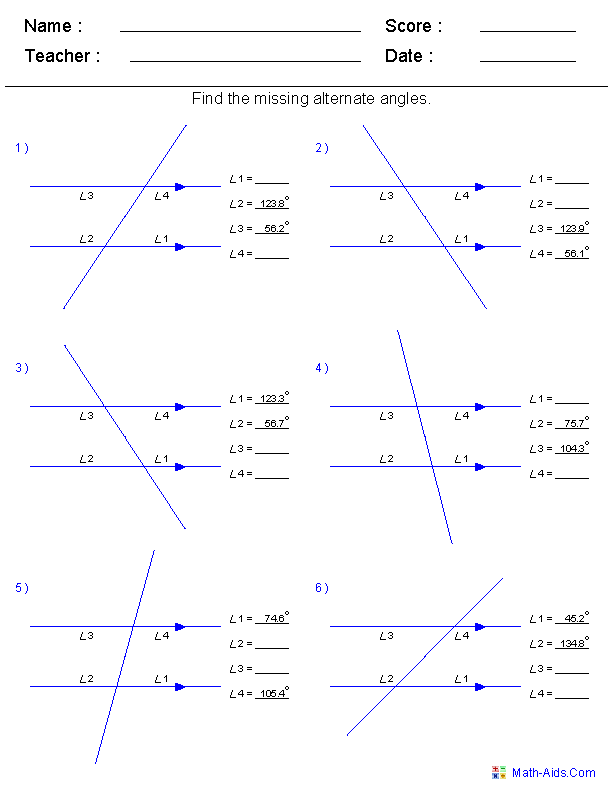##### Find Corresponding AnglesAngles Worksheets##### Find All AnglesAngles Worksheets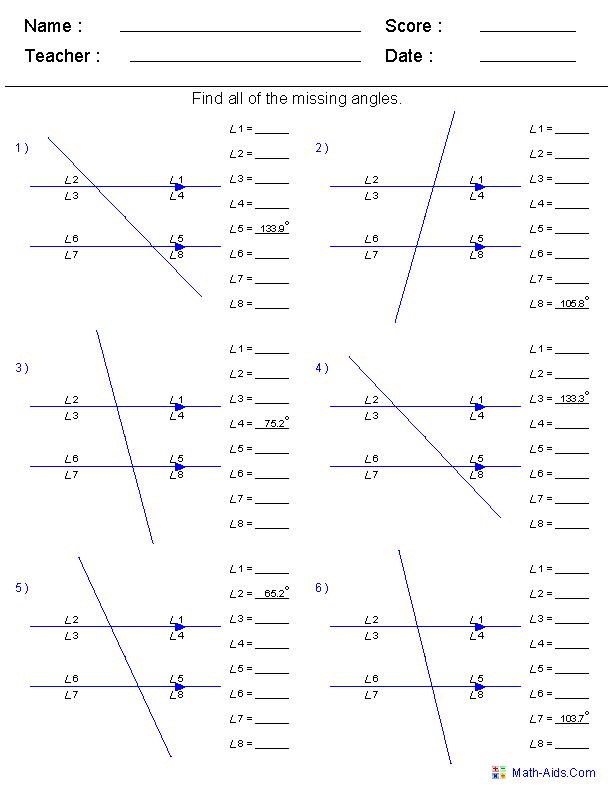##### Arcs and CentralAngles Worksheets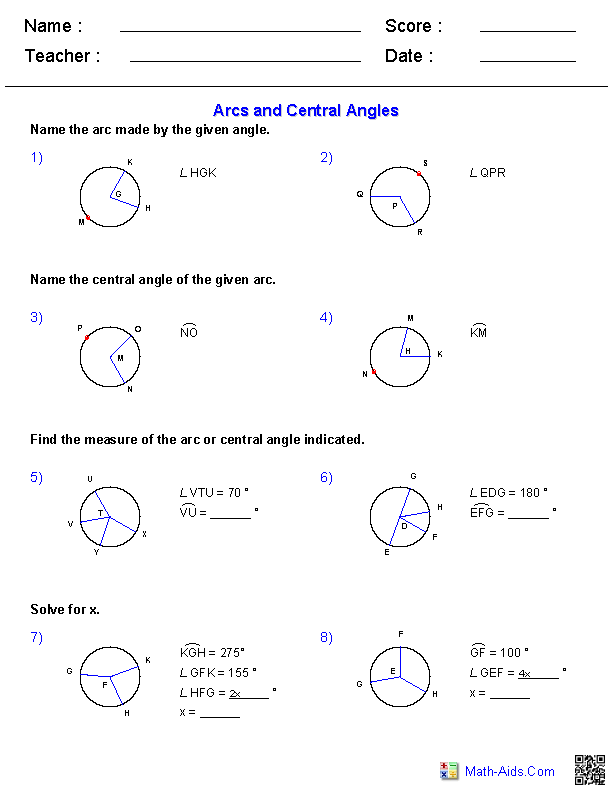##### Inscribed AnglesAngles WorksheetsRecommended Videos

## Detailed Description for All Angles Worksheets

Classifying Angles Worksheets
These Angles Worksheets are great for teaching the different classification of angles. These angles worksheets will produce 20 problems for the student to identify whether the angle is acute, obtuse, right, or straight.

Naming Angles Worksheets
These Angles Worksheets are great for teaching the correct nomenclature to identify angles and sides of angles. They will be asked to label the vertex and sides of angles and name all angles with a given vetex. These angles worksheets will produce 12 problems.

Angle Pair Relationships Worksheets
These Angles Worksheets are great for identifying angle pair relationships. The student will identify adjacent, complementary, linear pair, or vertical angles. These worksheets will produce 8 problems per page.

Producing Protractor Images Worksheets
These Angles Worksheets are great for handouts or overhead projector where a protractor image is needed. You may also print this protractor images on a piece of acetate to make a large size protractor for use in the classroom. These Angles Worksheets will produce one, two, four, or six images of a protractor per page.

Reading a Protractor Worksheets
These Angles Worksheets are perfect for practicing reading and using a protractor to measure different angles. These Angles Worksheets will produce two problems per page.

Measuring Angles Worksheets
These Angles Worksheets are great for practicing measuring angles with a protractor.
These worksheets will produce 8 problems per page.

Drawing Angles Worksheets
These Angles Worksheets are great for practicing drawing angles with a protractor.
These worksheets will produce 8 problems per page.

Identify if a Point is Interior or Exterior to an Angle Worksheets
These Angles Worksheets are great for practicing identifying if a point is interior, exterior, or on the angle.
These worksheets will produce 9 problems per page.

Angle Addition Postulate Worksheets
These Angles Worksheets are great for practicing the angle addition postulate.
These angle worksheets will produce 9 problems per page.

Find Complementary Angles Worksheets
These Angles Worksheets are great for practicing finding missing angles from complementary angle pairs.
You may select whole numbers or decimal numbers for the problems and configure the worksheet for 9, 12 or 15 problems.

Find Supplementary Angles Worksheets
These Angles Worksheets are great for practicing finding missing angles from supplementary angle pairs.
You may select whole numbers or decimal numbers for the problems and configure the worksheet for 6, 8 or 10 problems.

Find Vertical Angles Worksheets
These Angles Worksheets are great for practicing finding missing vertical angles from vertical angle pairs.
You may select whole numbers or decimal numbers for the problems and configure the worksheet for 6 or 8 problems.

Find Alternate Angles Worksheets
These Angles Worksheets are great for practicing finding missing alternate angles from various graphs.
You may select whole numbers or decimal numbers for the 6 problems that are generated per worksheet.

Find Corresponding Angles Worksheets
These Angles Worksheets are great for practicing finding missing corresponding angles from various graphs.
You may select whole numbers or decimal numbers for the 6 problems that are generated per worksheet.

Find All Angles Worksheets
These Angles Worksheets are great for practicing finding missing angles on a graph using complementary, supplementary, vertical, alternate, and corresponding angle relationships.
You may select whole numbers or decimal numbers for the 6 problems that are generated per worksheet.

Arcs and Central Angles Worksheets
These Angles Worksheets will produce problems for identifying and working with inscribed angles and arcs. You may select which figures to name, the number of points on the circle's perimeter, as well as the types of figures inscribed in the circles.

Inscribed Angles Angles Worksheets
These Angles Worksheets will produce problems for identifying and working with inscribed angles and arcs. You may select which figures to name, as well as the types of figures inscribed in the circles.